# CURRENT DIVISION

### CURRENT DIVIDER FORMULAClick or Tap the Example circuits below to invoke TINACloud and select the Interactive DC mode to Analyze them Online.
Get a low cost access to TINACloud to edit the examples or create your own circuitsVOLTAGE DIVISION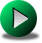POWER IN DC CIRCUITS

Using Ohm's law, we can derive a formula (called the current divider) that can be used to calculate the current through any branch of a multiple-branch parallel circuit: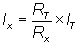where

Ix = current to be calculated, in branch x

RT= total resistance

Rx = resistance in branch x

IT = total current

Example 1

First, we'll solve for the currents using Ohm's Law.

Click/tap the circuit above to analyze on-line or click this link to Save under Windows

The total resistance: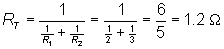The voltage:

V = RT*IS = 1.2*5 = 6 V;

and so the currents:Now let's see how to use the current divider formula. Although at first glance it looks as though we're using a different formula from the one given at the beginning of this tutorial, in fact the formula is equivalent for the case of two resistors in parallel. Starting from the formula given earlier, substitute (R1*R2)/(R1+R2) for Rt and simplify to arrive at the formula used below.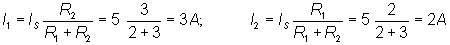The results are the same as those calculated by TINA.

Example 2

Find the current in resistor R1.

Click/tap the circuit above to analyze on-line or click this link to Save under Windows

Here we have two branches in parallel connected to the current source. One of the branches is a series-parallel circuit. Current division must be used twice: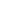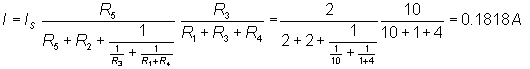VOLTAGE DIVISIONPOWER IN DC CIRCUITS